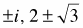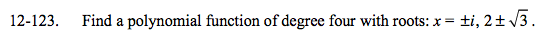### Home > A2C > Chapter 12 > Lesson 12.3.1 > Problem12-123

12-123.

Find a polynomial function of degree four with roots:. Homework Help ✎Use the Zero Product Property to write a product of the roots, then simplify.

$P(x)=(x+i)(x-i)(x-2+\sqrt{3})(x-2-\sqrt{3})=?$